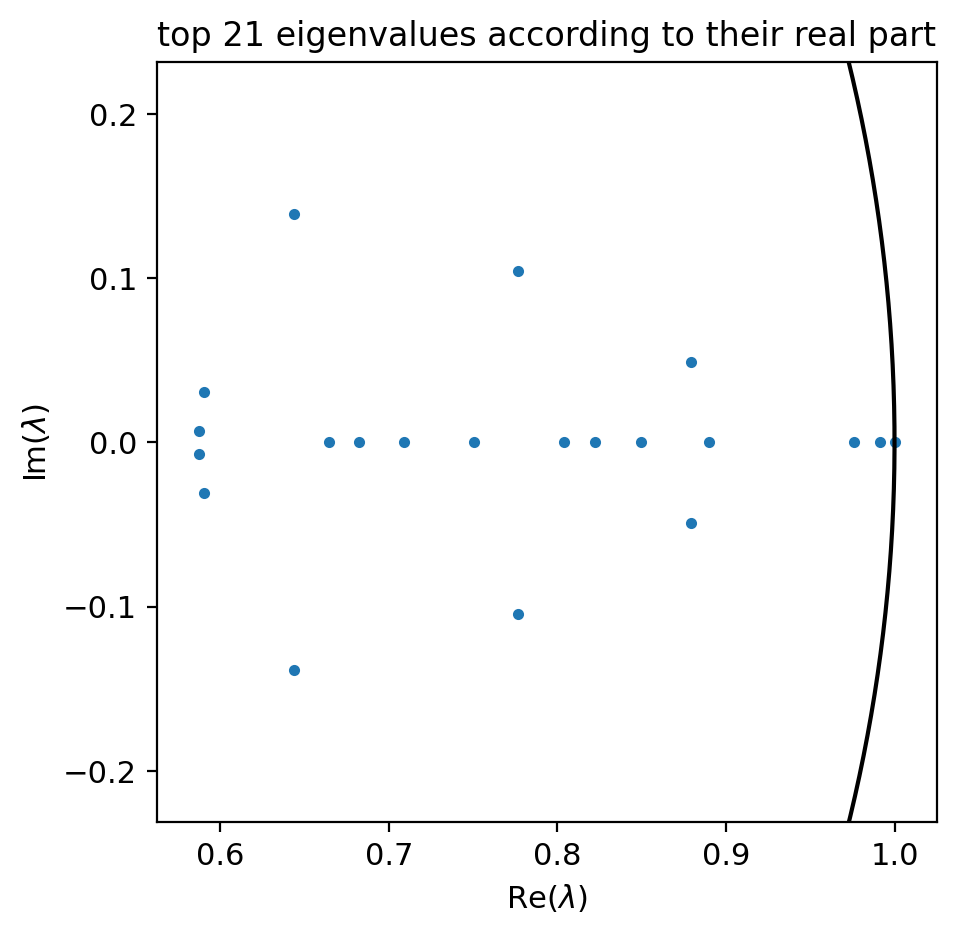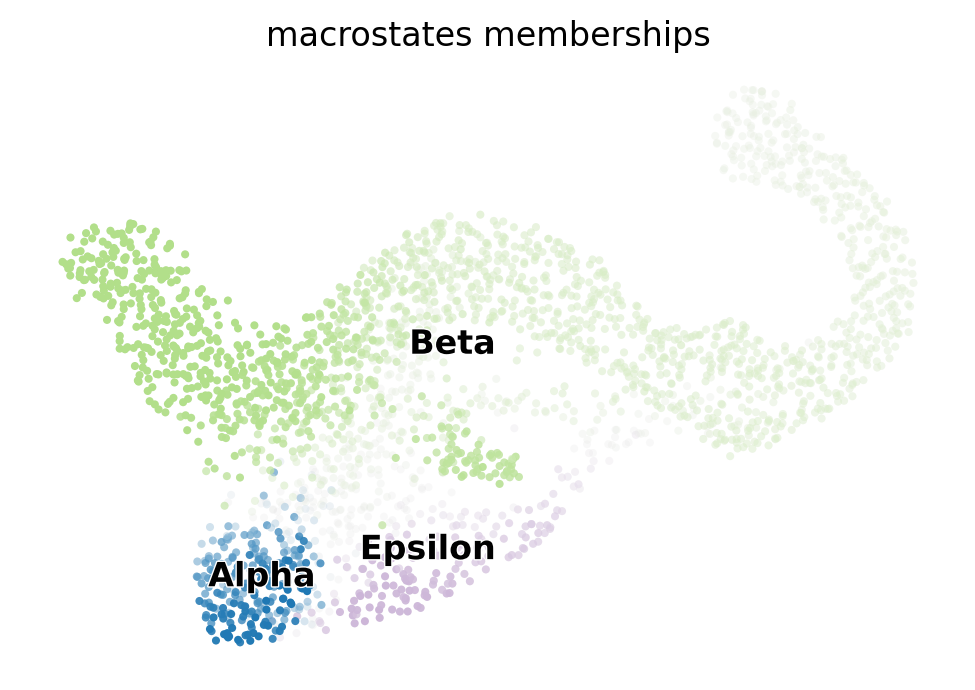# Kernels and estimators¶

This tutorial shows how to work with CellRank using the low-level mode. We will interact directly with CellRank's two main modules, kernels and estimators. Don't worry - this really isn't any more complicated than using scikit-learn.

We'll demonstrate this using a dataset of mouse pancreatic development at E15.5 Bastidas-Ponce et al., Development 2019. We'll use scVelo a bit to work with RNA velocity, if you're unfamiliar with that, see the documentation or read Bergen et al., Nat. Biotechnol. 2020.

## Concept of kernels and estimators¶The idea behind CellRank is to have a flexible and extensible scanpy/AnnData compatible modeling framework for single-cell data that resolves around the concept of Markov chains. Each state in the Markov chain is given by one observed cell and transition probabilities among cells only depend on the current cell and not on the history. To make CellRank as flexible as possible, we modularized it into kernels which compute a cell-cell transition matrix and estimators which use the transition matrix formulate hypotheses about the underlying dynamics, see the above figure.

Kernels read data from an AnnData object like RNA velocity vectors, transcriptomic similarity, spatial proximity, pseudotime, etc. and use it to compute a sparse transition matrix (see figure above). We set up the kernel structure so that it's easy for you to contribute your own kernel via CellRank's external module, check out our create your own kernel tutorial.

Estimators read a cell-cell transition matrix from a kernel object and compute aggregate dynamics based on it. Each estimator works with every kernel - the core idea here is that it does not matter how you computed your transition matrix to run an estimator on top, you can even compute a transition matrix outside of CellRank and import it via a PrecomputedKernel - you will still be able to use every estimator on top. Our main estimator is called Generalized Perron Cluster Cluster Analysis (GPCCA) and we interface to the great pyGPCCA library for it, see Reuter et al., JCTC 2018 and Reuter et al., JCP 2019.

Besides kernels and estimators, there are downstream plotting functions that use fate probabilities to do fun stuff, i.e. giving you a directed version of PAGA, plotting gene expression trends along lineages or embedding cells in a circle based on where they're likely to go - to name only a few. Check out CellRank's API and our gallery for a full list.

This tutorial will focus on getting you familiar with the structure of kernels and estimators, how they can be used to set up and analyze a single-cell Markov chain and how you can extract real biological knowledge from them. If you would like to learn something about the math behind them, or see more biological applications, check out the CellRank preprint Lange et al., bioRxiv 2020. Enjoy the tutorial!

## Import packages & data¶

Easiest way to start is to download Miniconda3 along with the environment file found here. To create the environment, run conda create -f environment.yml.

In :
import sys

!pip install -q git+https://github.com/theislab/[email protected]

In :
import scvelo as scv
import scanpy as sc
import cellrank as cr
import numpy as np

scv.settings.verbosity = 3
scv.settings.set_figure_params("scvelo")
cr.settings.verbosity = 2


We'll not go trough all the pre-processing steps described in the basic tutorial again - instead, we will download an AnnData object that contains pre-processed (filtered, total counts normalized & log-transformed) data where RNA velocity has already been pre-computed for us. The following commands will download the pre-processed adata object and save it under datasets/endocrinogenesis_day15.5_preprocessed.h5ad. Beware - this object is about 150 MB large.

In :
adata = cr.datasets.pancreas_preprocessed()

Abundance of ['spliced', 'unspliced']: [0.81 0.19]

100%|██████████| 140M/140M [00:07<00:00, 18.8MB/s]

Out:
AnnData object with n_obs × n_vars = 2531 × 2000
obs: 'day', 'proliferation', 'G2M_score', 'S_score', 'phase', 'clusters_coarse', 'clusters', 'clusters_fine', 'louvain_Alpha', 'louvain_Beta', 'initial_size_unspliced', 'initial_size_spliced', 'initial_size', 'n_counts', 'velocity_self_transition', 'dpt_pseudotime'
var: 'highly_variable_genes', 'gene_count_corr', 'means', 'dispersions', 'dispersions_norm', 'fit_r2', 'fit_alpha', 'fit_beta', 'fit_gamma', 'fit_t_', 'fit_scaling', 'fit_std_u', 'fit_std_s', 'fit_likelihood', 'fit_u0', 'fit_s0', 'fit_pval_steady', 'fit_steady_u', 'fit_steady_s', 'fit_variance', 'fit_alignment_scaling', 'velocity_genes'
uns: 'clusters_colors', 'clusters_fine_colors', 'diffmap_evals', 'iroot', 'louvain_Alpha_colors', 'louvain_Beta_colors', 'neighbors', 'pca', 'recover_dynamics', 'velocity_graph', 'velocity_graph_neg', 'velocity_params'
obsm: 'X_diffmap', 'X_pca', 'X_umap', 'velocity_umap'
varm: 'PCs', 'loss'
layers: 'Ms', 'Mu', 'fit_t', 'fit_tau', 'fit_tau_', 'spliced', 'unspliced', 'velocity', 'velocity_u'
obsp: 'connectivities', 'distances'

## Initialize a kernel¶

To construct a transition matrix, CellRank offers a number of kernel classes in cellrank.tl.kernels. To demonstrate the concept, we'll restrict our attention to the following two kernels here:

• VelocityKernel: compute transition matrix based on RNA velocity.
• ConnectivityKernel: compute symmetric transition matrix based on transcriptomic similarity - essentially a diffusion-pseudotime (DPT) kernel Haghverdi et al., Nature Methods 2016.

For a full list of kernels, check out our API. To initialize a kernel object, simply run the following:

In :
from cellrank.tl.kernels import VelocityKernel



Note that kernels need an AnnData object to read data from it - CellRank is part of the Scanpy/AnnData ecosystem. The only exception to this is the PrecomputedKernel which directly accepts a transition matrix, thus making it possible to interface to CellRank from outside the scanpy/AnnData world.

In :
print(vk)

<VelocityKernel>


There isn't very much here yet!

## Compute a transition matrix¶

To compute a transition matrix, every kernel object has a corresponding method:

In :
vk.compute_transition_matrix()

Computing transition matrix based on logits using 'deterministic' mode
Estimating softmax_scale using 'deterministic' mode
Setting softmax_scale=3.9741
Finish (0:00:04)

100%|██████████| 2531/2531 [00:01<00:00, 1303.87cell/s]
100%|██████████| 2531/2531 [00:01<00:00, 1440.28cell/s]

Out:
<VelocityKernel>

Which algorithm is run under the hood to get the cell-cell transition probabilities depends entirely on the kernel - in this case, we compared RNA velocity vectors to transcriptomic displacements in local neighborhoods to estimate where each single cell is likely to go. To see how exactly this transition matrix was computed, we can print the kernel again:

In :
print(vk)

<VelocityKernel[softmax_scale=3.97, mode=deterministic, seed=45795, scheme=<CorrelationScheme>]>


There's a lot more info now! To find out what all of these mean, check the docstring of .compute_transition_matrix. The most important bits of information here are:

• mode='deterministic: by default, the computation is deterministic, but we can also sample from the velocity distribution (mode='sampling'), get a 2nd order estimate (mode='stochastic') or a Monte Carlo estimate (mode='monte_carlo').
• backward=False: run the process in the forward direction. To change this, set backward=True when initializing the VelocityKernel.
• softmax_scale: scaling factor used in the softmax to transform cosine similarities into probabilities. The larger this value, the more centered the distribution will be around the most likely cell. If None, use velocity variances to scale the softmax, i.e. an automatic way to tune it in terms of local variance in velocities. This requires one additional run (always in 'deterministic' mode, to quickly estimate the scale).

## Combining two kernels¶

RNA velocity vectors can sometimes be very noisy - to regularize them, let's combine the VelocityKernel with a ConnectivityKernel, computed on the basis of transcriptomic similarity. Let's start by initializing such a kernel and computing a second transition matrix:

In :
from cellrank.tl.kernels import ConnectivityKernel


Computing transition matrix based on adata.obsp['connectivities']
Finish (0:00:00)


The ConnectivityKernel has a parameter conn_key which is used to read the KNN graph from adata.obsp. By default, this is set to connectivities, meaning that a transcriptomic similarity-based KNN graph is used. If you have spatial data and you've processed in using squidpy Palla et al., bioRxiv 2021, you can read a spatial-proximity based KNN graph by varying the conn_key to whatever was used to save the graph in squidpy, by default, spatial_connectivities.

Note how it's possible to call the .compute_transition_matrix method direcly when initializing the kernel - this works for all kernel classes. Given these two kernels now, we can combine them with some relative weights:

In :
combined_kernel = 0.8 * vk + 0.2 * ck


Let's print the combined_kernel to see what happened:

In :
print(combined_kernel)

((0.8 * <VelocityKernel[softmax_scale=3.97, mode=deterministic, seed=45795, scheme=<CorrelationScheme>]>) + (0.2 * <ConnectivityKernel[dnorm=True, key=connectivities]>))


There we go, we took the two computed transition matrices stored in the kernel object and combined them using a weighted mean, with weights given by the factors we provided. We will use the combined_kernel in the estimators section below.

## Initialize an estimator¶

Estimators take a kernel object and offer methods to analyze it. The main objective is to decompose the state space into a set of macrostates that represent the slow-time scale dynamics of the process. A subset of these macrostates will be the initial or terminal states of the process, the remaining states will be intermediate transient states. CellRank currently offers two estimator classes in cellrank.tl.estimators:

• CFLARE: Clustering and Filtering Left And Right Eigenvectors. Heuristic method based on the spectrum of the transition matrix.
• GPCCA: Generalized Perron Cluster Cluster Analysis: project the Markov chain onto a small set of macrostates using a Galerkin projection which maximizes the self-transition probability for the macrostates, see Reuter et al., JCTC 2018 and Reuter et al., JCP 2019.

For more information on the estimators, have a look at the API. We will demonstrate the GPCCA estimator here, however, the CFLARE estimator has a similar set of methods (which do different things internally). Let's start by initializing a GPCCA object based on the combined_kernel we constructed above:

In :
from cellrank.tl.estimators import GPCCA

g = GPCCA(combined_kernel)
print(g)

GPCCA[n=2531, kernel=((0.8 * <VelocityKernel[softmax_scale=3.97, mode=deterministic, seed=45795, scheme=<CorrelationScheme>]>) + (0.2 * <ConnectivityKernel[dnorm=True, key=connectivities]>))]


Additionally to the information about the kernel it is based on, this prints out the number of states in the underlying Markov chain.

## Compute a matrix decomposition¶

GPCCA needs a real sorted Schur decomposition to work with, so let's start by computing this and visualizing eigenvalues in complex plane:

In :
g.compute_schur(n_components=20)
g.plot_spectrum()

WARNING: Unable to import petsc4py or slepc4py. Using method='brandts'
WARNING: For method='brandts', dense matrix is required. Densifying
Computing Schur decomposition
WARNING: Using 20 components would split a block of complex conjugate eigenvalues. Using n_components=21
When computing macrostates, choose a number of states NOT in [5, 10, 16, 18, 20]
Adding adata.uns['eigendecomposition_fwd']
.schur_vectors
.schur_matrix
.eigendecomposition
Finish (0:00:21)To compute the Schur decomposition, there are two methods implemented:

• method='brandts': use scipy.linalg.schur to compute a full real Schur decomposition and sort it Brandts, Numerical linear algebra with applications 2002. Note that scipy.linalg.schur only supports dense matrices, so consider using this for small cell numbers (<10k).
• method='krylov': use an iterative, krylov-subspace based algorithm provided in SLEPc to directly compute a partial, sorted, real Schur decomposition. This works with sparse matrices and will scale to extremely large cell numbers.

The real Schur decomposition for transition matrix T is given by Q U Q**(-1), where Q is orthogonal and U is quasi-upper triangular, which means it's upper triangular except for 2x2 blocks on the diagonal. 1x1 blocks on the diagonal represent real eigenvalues, 2x2 blocks on the diagonal represent complex eigenvalues. Above, we plotted the top 20 eigenvalues of the matrix T to see whether there is an apparent eigengap. In the present case, there seems to be such a gap after the first 3 eigenvalues.

Schur vectors will span an invariant subspace, let's call it X (Schur vectors in the columns). Note that the Schur decomposition is not unique - however, the subspace spanned by the top Schur vectors is unique so will be our initial & terminal states as well as fate probabilities.

## Infer terminal states¶

The next step in GPCCA is to find a linear combination of these vectors such that the Markov chain defined on the subset of states has large self-transition probability. We do this by calling the following method:

In :
g.compute_macrostates(n_states=3, cluster_key="clusters")
g.plot_macrostates()

Computing 3 macrostates
Adding .macrostates
.macrostates_memberships
.coarse_T
.coarse_initial_distribution
.coarse_stationary_distribution
.schur_vectors
.schur_matrix
.eigendecomposition
Finish (0:00:00)We can look at individual states by passing same_plot=False:

In :
g.plot_macrostates(same_plot=False)
`